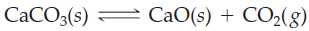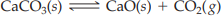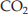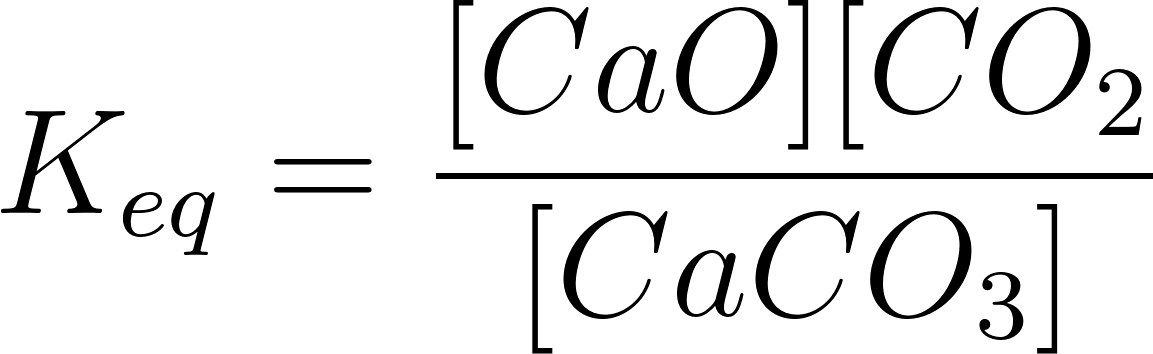×
Get Full Access to Introductory Chemistry - 5 Edition - Chapter 15 - Problem 56p
Get Full Access to Introductory Chemistry - 5 Edition - Chapter 15 - Problem 56p

×

# Answer: Consider the reaction. An equilibrium mixture of this reaction at a certainISBN: 9780321910295 34

## Solution for problem 56P Chapter 15

Introductory Chemistry | 5th Edition

• Textbook Solutions
• 2901 Step-by-step solutions solved by professors and subject experts
• Get 24/7 help from StudySoup virtual teaching assistantsIntroductory Chemistry | 5th Edition

4 5 1 394 Reviews
15
2
Problem 56P

Consider the reaction.An equilibrium mixture of this reaction at a certain temperature has [CO2] = 0.548 M. What is the value of the equilibrium constant at this temperature?

Step-by-Step Solution:

Problem 56P

Consider the reaction.An equilibrium mixture of this reaction at a certain temperature has [] = 0.548 M. What is the value of the equilibrium constant at this temperature?

Step by Step Solution

Step 1 of 2

The given reaction is as follows.Let’s write the equilibrium constant for the given balanced reaction....................(1)

Step 2 of 2

##### ISBN: 9780321910295

This full solution covers the following key subjects: temperature, reaction, Equilibrium, mixture, constant. This expansive textbook survival guide covers 19 chapters, and 2046 solutions. Since the solution to 56P from 15 chapter was answered, more than 392 students have viewed the full step-by-step answer. Introductory Chemistry was written by and is associated to the ISBN: 9780321910295. This textbook survival guide was created for the textbook: Introductory Chemistry, edition: 5. The answer to “?Consider the reaction. An equilibrium mixture of this reaction at a certain temperature has [CO2] = 0.548 M. What is the value of the equilibrium constant at this temperature?” is broken down into a number of easy to follow steps, and 29 words. The full step-by-step solution to problem: 56P from chapter: 15 was answered by , our top Chemistry solution expert on 05/06/17, 06:45PM.

Unlock Textbook Solution